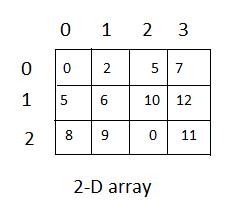# C# Multidimensional array

In the previous chapter, we learned about C# arrays, how to initialize array and declare array in C#, now in this tutorial, we will see how to create multidimensional 2-D array in C#. We declare a one-dimensional array of integer numbers using `int[]`, and we declare a two-dimensional with `int[,]`. For example :

``int[,] twoDimensionalArray;``

Above array has two dimensions, that is why it is called as 2 Dimensional array, while has it has more than one dimension, it is also called as multi-dimensional array in C#, these are also called as Matrices in mathematical term.

Similarly, we can declare three-dimensional array as

``int[,,] threeDimensionalArray;``

Syntax for declaring Multi-dimensional array in C#

``````dataType[,] variableName;         //  Two dimensional array
dataType[,,] variableName;       //   Three dimensional array

dataType[,] variableName = new dataType[size,size];            //  Three dimensional array
dataType[,,] variableName = new dataType[size,size,size];     //   Three dimensional array``````

Where,

datatype = can be any datatype, which will be stored in the array, can be int, string, char etc.

variableName = name of the array

size= size of row/column in an array

### Multidimensional array declaration

We can declare multidimensional array in same way as we single dimension arrays, we just need to add comma (,) inside square brackets, for example

``````int[,] intMatrixArray; // for 2-d array, use one comma
string[,,] strCubeArray; // for 3-d array, using two comma``````

In the above examples, we have created two-dimensional int array and three-dimensional string array, each dimension is represented by comma(,).

We can allocate memory(size) of multidimensional array by using `new` keyword, as shown below

``````int[,] intMatrixArray = new int[3, 4];
string[,,] strCubeArray = new string[5, 5, 5];``````

In the above 2-D array, we are allocating size of 3 rows and 4 columns, take a look at the image below for understanding it### Multi-Dimensional Array Initialization

We can initialize 2-D array as shown below

``````int[,] matrix = {
{0, 2, 4, 8}, // row 0 values
{10, 12, 14, 16}, // row 1 values
};``````

OR

``````int[,] array= new int[4,2] {
{ 1, 2 },
{ 3, 4 },
{ 5, 6 },
{ 7, 8 }
};``````

for 3-D array, we can initialize it as shown  below

``````int[,,] array= {
{{12, 2, 8}},
{{14, 5, 2}},
{{3, 26, 9}},
{{4, 11, 2}}
};``````

### Example of multi dimensional array in C#

#### Two dimensional array in C# example

``````using System;

public class Program
{
public static void Main()
{
//two dimensional array declared and initiliazed
int row=2;
int columns=4;
int[,] matrix = new int[2,4]{
{0, 2, 4, 8}, // row 0 values
{10, 12, 14, 16}, // row 1 values
};

for (int i=0; i<row; i++)
{
for (int j=0; j<columns; j++)
{
Console.WriteLine(" Row "+i+ " , column "+j +" value ="+matrix[i, j]);
}
}
}
}``````

Output

`````` Row 0 , column 0 value =0
Row 0 , column 1 value =2
Row 0 , column 2 value =4
Row 0 , column 3 value =8
Row 1 , column 0 value =10
Row 1 , column 1 value =12
Row 1 , column 2 value =14
Row 1 , column 3 value =16``````

#### Three dimensional array example in C#

``````using System;

public class ThreeDimensionalArrayProgram
{
public static void Main()
{
//three dimensional array
int[,,] array= {
{{12, 2, 8}},
{{14, 5, 2}},
{{3, 26, 9}},
{{4, 11, 2}}
};
//get array lengths
int d1 = array.GetLength(0); // array length at 1st dimension
int d2 = array.GetLength(1); // array length at 2nd dimension
int d3 = array.GetLength(2); // array length at 3rd dimension

for (int i=0; i<d1; i++)
{
for (int j=0; j<d2; j++)
{
for (int k=0; k<d3; k++)
{
Console.WriteLine(" value at position "+i+ " "+j+" "+k+ " ="+array[i, j, k]);
}
}
}

}
}``````

output

``````value at position 0 0 0 =12
value at position 0 0 1 =2
value at position 0 0 2 =8
value at position 1 0 0 =14
value at position 1 0 1 =5
value at position 1 0 2 =2
value at position 2 0 0 =3
value at position 2 0 1 =26
value at position 2 0 2 =9
value at position 3 0 0 =4
value at position 3 0 1 =11
value at position 3 0 2 =2``````

In the above code, we are using `array.GetLength(Dimension)` method, which gives us size of array, it is useful to get the number of elements in the specified dimension of the array.

### Accessing Array Elements in MultiDimensional Array

We can directly access array element, by passing it's index, for two dimensional array, you need to pass two index's, first index = row and second index = column number, for example:

``````int[,] myArray = new int[3,2]{
{1, 2},
{3, 4},
{5, 6}
};

myArray [0,0]; //Output: 1
myArray [0,1]; // 2

myArray [1,0]; // 3
myArray [1,1]; // 4

myArray [2,0]; // 5
myArray [2,1]; // 6

``````

Similarly, for three dimensional array, you need to pass all three indexes locations

``````using System;

public class Program
{
public static void Main()
{
int[,,] array= {
{{12, 2, 8}},
{{14, 5, 2}},
{{3, 26, 9}},
{{4, 11, 2}}
};

Console.WriteLine(array[0,0,0]); //outputs 12
Console.WriteLine(array[0,0,1]); // outputs 2
Console.WriteLine(array[0,0,2]); //outputs 8
Console.WriteLine(array[1,0,0]);//outputs 14
}
}``````

Output

``````12
2
8
14``````

Similarly, you are access all locations by passing indexes to an array.